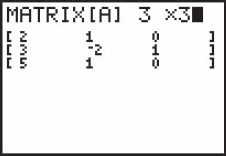### 10.4. Higher-Order Determinants

To solve a system of more than two equations by determinants, we must be able to write and evaluate determinants of orders greater than 2. We show how to do that here, first by calculator and then by the use of minors. The calculator method is, of course, the fastest and easiest but can only be used with equations that have numerical coefficients. The method of minors will work for literal equations as well as numerical.

#### 10.4.1. Evaluating a Higher-Order Determinant by Calculator

The procedure for evaluating a higher-order determinant by calculator is no different from that for a second-order determinant.

## Example 23:

Evaluate the determinantSolution: The calculator screens are as shown.

TI-83/84 screens for Example 23.

#### 10.4.2. Development by Minors

The following method may be used to evaluate determinants of any size. It is more difficult than using a calculator but can be used for determinants that contain letters.

The minor of an element in a determinant is a determinant of next lower order, obtained by deleting the row and the column in which that element lies.(1) In theEDIT screen we enter the matrix dimensions and the nine elements.

Get Technical Mathematics, Sixth Edition now with the O’Reilly learning platform.

O’Reilly members experience live online training, plus books, videos, and digital content from nearly 200 publishers.